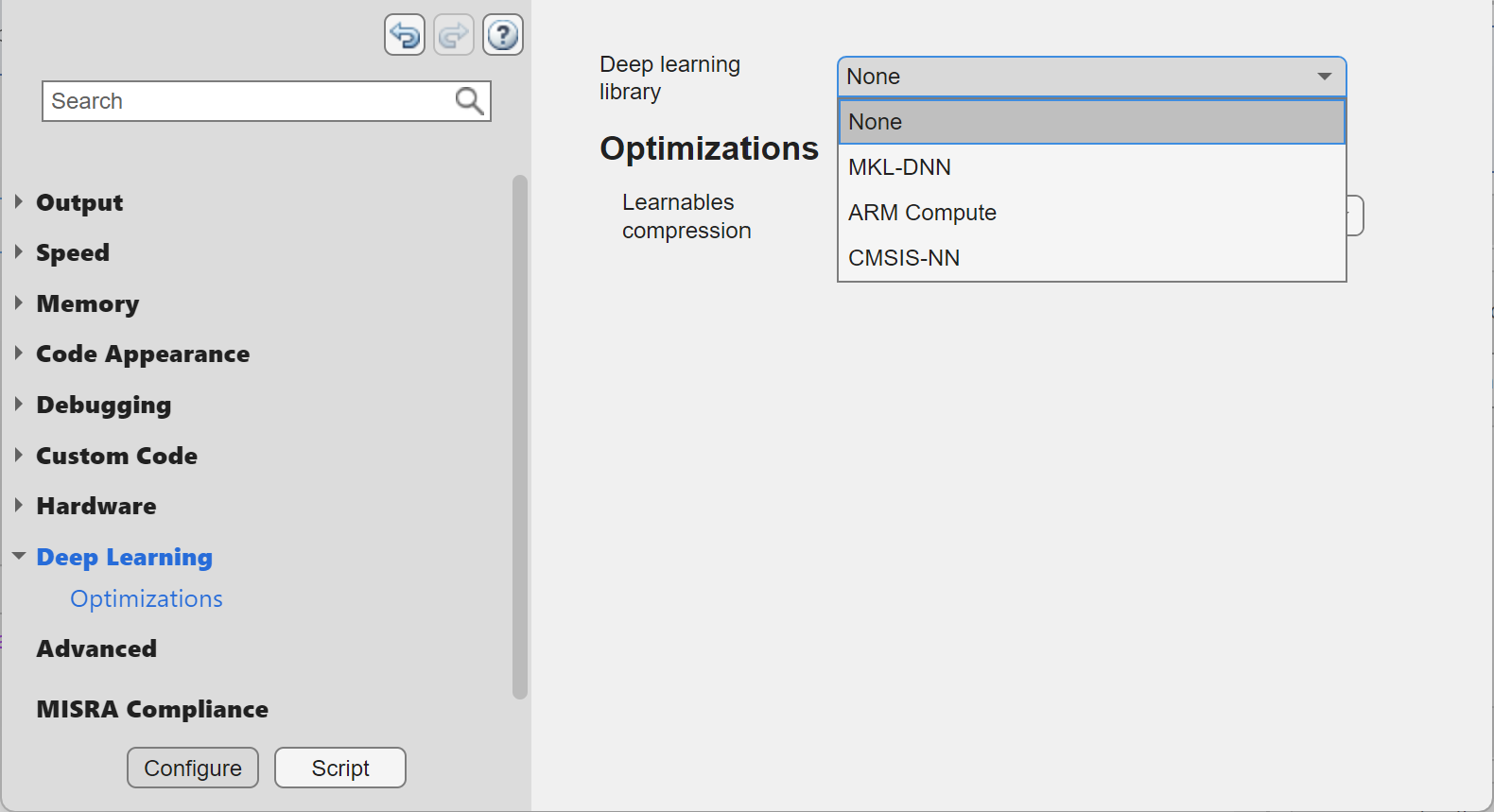## Generate Generic C/C++ Code for Deep Learning Networks

With MATLAB® Coder™, you can generate generic C or C++ code for prediction from an already trained convolutional neural network (CNN). The generated C/C++ code does not depend on any third-party libraries. The generated code implements a CNN with the architecture, layers, and parameters specified in the input `SeriesNetwork` (Deep Learning Toolbox) or `DAGNetwork` (Deep Learning Toolbox) network object. See Networks and Layers Supported for Code Generation.

Generate code by using one of these methods:

• The standard `codegen` command for C/C++ code generation from MATLAB code.

• The MATLAB Coder app.

### Requirements

• On Windows®, code generation for deep learning networks with the `codegen` function requires Microsoft® Visual Studio® or the MinGW® compiler.

• MATLAB Coder Interface for Deep Learning Libraries. To install this support package, select it from the MATLAB Add-Ons menu.

• Deep Learning Toolbox™.

### Code Generation by Using `codegen`

1. Write an entry-point function in MATLAB that:

For example:

```function out = my_predict(in) %#codegen % A persistent object mynet is used to load the series network object. % At the first call to this function, the persistent object is constructed and % setup. When the function is called subsequent times, the same object is reused % to call predict on inputs, thus avoiding reconstructing and reloading the % network object. persistent mynet; if isempty(mynet) mynet = coder.loadDeepLearningNetwork('myNetwork.mat'); end % pass in input out = predict(mynet,in,'MiniBatchSize',2); ```

2. Create a deep learning configuration object `dlconfig` that is configured for generating generic C/C++ code by using the `coder.DeepLearningConfig` function.

`dlconfig = coder.DeepLearningConfig(TargetLibrary='none');`

Create a code generation configuration object for MEX or for a static or dynamically linked library. By default, the code generator produces generic C code. To produce generic C++ code, in your code generation configuration object, set the `TargetLang` parameter to `'C++'`. Set the `DeepLearningConfig` parameter to the previously created object `dlconfig`.

```cfg = coder.config('lib'); cfg.TargetLang = 'C++'; cfg.DeepLearningConfig = dlconfig;```
3. Run the `codegen` command. Use the `-config` option to specify the configuration object. Use the `-args` option to specify the input type.

`codegen -config cfg my_predict -args {myInput} -report`

Note

You can specify half-precision inputs for code generation. However, the code generator type casts the inputs to single-precision. The Deep Learning Toolbox uses single-precision, floating-point arithmetic for all computations in MATLAB.

### Code Generation by Using the MATLAB Coder App

1. Follow the usual steps for specifying the entry-point function and specifying input types. See Generate C Code by Using the MATLAB Coder App.

2. In the Generate Code step:

• Set Language to either C or C++.

• Click More Settings. In the Deep Learning pane, set Target library to `None`.3. Generate code.